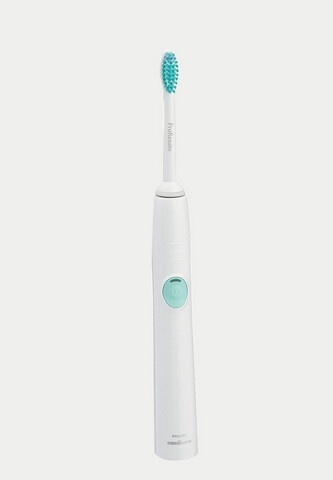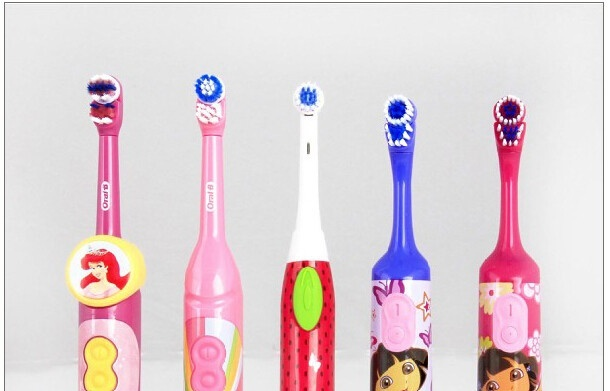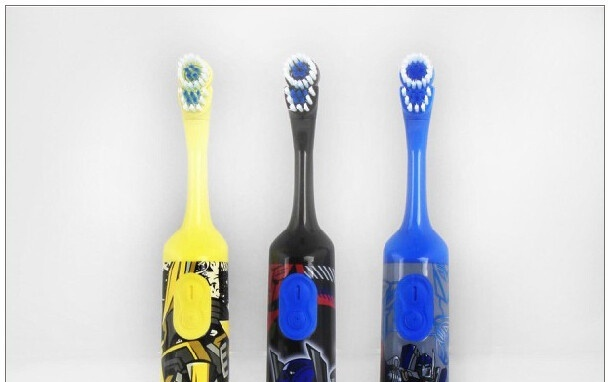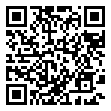|

# 小常识 你的牙齿适合哪种刷牙方法

刷牙是保持口腔清洁的主要方法，它能消除口腔内软白污物、食物碎片和部分牙面菌斑，而且有按摩牙龈作用，从而减少口腔环境中致病因素，增强组织的抗病能力，刷牙对于预防各种口腔疾病，特别是对于预防和治疗牙周病和龋病等，具有重要的作用。刷牙的方法

刷牙的方法种类很多，有些方法既合理又方便，值得介绍与推广，每一种方法有它一定的特点，也仅适用于不同年龄和不同个体情况，没有一种刷牙法能适合于所有的人。人群中习惯采用的横刷法弊病较多，但如予以改进，也可变成一种较好的刷牙方法。任何一种好的刷牙法应当简单易学，清洁牙齿效果好，不损伤牙体和牙周组织。实际上，人们能否良好地掌握刷牙方法，很大程度上取决于双手的灵活性。虽然每个人刷牙的手势有巧拙之分，但只要给予充分诱导，一般人都能有效地使用牙刷清洁口腔。儿童的动作比较迟缓，且缺乏耐性，不能应付复杂的刷牙技巧，应教他们使用比较简单的刷牙方法。至于伤残人士，可能需要采用刷柄设计经过修改的牙刷，方便抓握，或使用电动牙刷。刷牙方法与牙刷的品种有非常密切的关系，这里主要介绍普通牙刷的刷牙方法。

生理刷牙方法：将牙刷毛与牙面接触，刷毛顶端指向冠方，然后沿牙面向牙龈轻微拂刷，类似咀嚼纤维性食物对牙面的摩擦动作。这种方法能清洁牙面和刺激牙龈组织的血液循环。增进牙周组织健康。

比斯刷牙法洗刷唇舌面时，刷毛与牙面呈45度角，刷毛头指向牙龈方向，使刷毛进入龈沟和邻间区、部分刷毛压于龈缘上作前后向短距离来回颤动。刷毛紧压在面，使毛端深入裂沟区作短距离的前后向颤动。这种方法由于清洁能力较强，克服了拉锯式的横刷法的缺点，而变为短横刷，能有效地除去颈部及龈沟内的菌斑，按摩牙龈，还可避免，造成牙颈部楔状缺损及牙龈萎缩。

旋转式刷牙法 (roll method)：从牙龈往牙冠方向旋转刷。刷前牙唇面、后牙颊面 和后牙舌腭面时，牙刷毛束的尖端朝向牙龈，即上牙朝上，下牙朝下。牙刷毛与牙面呈45°角。第二步，将牙刷朝冠向做小环形旋转运动。第三步，顺牙缝刷洗，即可将各个牙面刷干净。刷前牙舌腭面时，牙刷毛束尖直接放在牙齿的舌腭面，上牙向下拉，下牙向上提，刷后牙咬合面时将牙毛放在咬面上，前后来回刷。刷牙技术要点：

1)辨认菌斑的附着部位：这是取得刷牙效果非常重要的环节。菌斑显示则是辨认菌斑的可靠方法。

2)刷毛紧贴牙面：口腔的解剖结构复杂，凹凸不平的区域较多，尤其是牙齿的邻接面和最后部磨牙的远中面，是最易堆积菌斑的部位。对其磨牙远中邻面刷洗时，从一个方向或从不同的方向、角度，尽量把刷毛伸进并与牙面紧贴，才可能对它作清刷。

3)牙刷头的动作：口腔结构较复杂，仅用一种刷牙方法，一个刷牙动作，是难以去净口内菌斑的。人们刷牙时，牙刷头的基本动作有纵向、横向、旋转和颤动四种，以完成刷牙过程。

4）顺序:上述各种刷牙方法，在熟练的基础上也可以综合起来，例如先作水平颤动法，然后要作几下垂直颤动法，择其优点，保持口腔卫生。横刷法有哪些危害？

我们不仅要养成良好的刷牙习惯，而且要讲究刷牙的方法。如果刷牙法不对头，也可造成流弊，最常见的损害是牙面耗损和牙龈萎缩。

横刷法是一般人群自发性的刷法，一种沿习最久、最普遍的刷法，其方法是将刷毛的尖端与牙齿表面接触，作向前向后拉锯式的刷牙动作。它的缺点很多，主要是不能适应口腔解剖形态上的要求，不能刷净牙齿，特别是难以洗刷牙齿的舌面和两牙之间的缝隙，也不能在到按摩牙龈的目的，而且容易引起牙体硬组织和牙龈的损伤。为此，我们应该坚决放弃这种不良的横刷法，而应采用正确的刷牙方法。横刷法对牙体硬组织的损害是牙质耗损主要特点有：由横刷法引起的牙质耗损性纹痕，多半在一群牙冠的唇颊面上，而且常为同等高度的平行线。若由横刷法引起的一群牙齿形成楔状缺损时，各牙缺陷的底线均在一条直线上。横刷法对口腔软组织可能引起的损害 ①牙龈和口腔粘膜发生擦伤、刺伤、或引起的炎症。②引起牙龈萎缩。牙龈在口腔内呈波浪形，牙龈边缘呈弧形较高，牙龈乳头则较低。若要刷净牙颈部，则牙龈乳头常被刷伤、时间久了就会出现牙龈萎缩。其特点是：唇颊侧的萎缩比舌侧重，牙弓突出的尖牙区颊侧受害更重，右手刷牙者，左侧的牙龈萎缩常较重。当停止使用横刷法后，牙龈受损的情况可逐渐好转。

`声明：本文由入驻焦点开放平台的作者撰写，除焦点官方账号外，观点仅代表作者本人，不代表焦点立场错误信息举报电话： 400-099-0099，邮箱：jubao@vip.sohu.com，或点此进行意见反馈，或点此进行举报投诉。`A B C D E F G H J K L M N P Q R S T W X Y Z
A - B - C - D - E
• A
• 鞍山
• 安庆
• 安阳
• 安顺
• 安康
• 澳门
• B
• 北京
• 保定
• 包头
• 巴彦淖尔
• 本溪
• 蚌埠
• 亳州
• 滨州
• 北海
• 百色
• 巴中
• 毕节
• 保山
• 宝鸡
• 白银
• 巴州
• C
• 承德
• 沧州
• 长治
• 赤峰
• 朝阳
• 长春
• 常州
• 滁州
• 池州
• 长沙
• 常德
• 郴州
• 潮州
• 崇左
• 重庆
• 成都
• 楚雄
• 昌都
• 慈溪
• 常熟
• D
• 大同
• 大连
• 丹东
• 大庆
• 东营
• 德州
• 东莞
• 德阳
• 达州
• 大理
• 德宏
• 定西
• 儋州
• 东平
• E
• 鄂尔多斯
• 鄂州
• 恩施
F - G - H - I - J
• F
• 抚顺
• 阜新
• 阜阳
• 福州
• 抚州
• 佛山
• 防城港
• G
• 赣州
• 广州
• 桂林
• 贵港
• 广元
• 广安
• 贵阳
• 固原
• H
• 邯郸
• 衡水
• 呼和浩特
• 呼伦贝尔
• 葫芦岛
• 哈尔滨
• 黑河
• 淮安
• 杭州
• 湖州
• 合肥
• 淮南
• 淮北
• 黄山
• 菏泽
• 鹤壁
• 黄石
• 黄冈
• 衡阳
• 怀化
• 惠州
• 河源
• 贺州
• 河池
• 海口
• 红河
• 汉中
• 海东
• I
• J
• 晋中
• 锦州
• 吉林
• 鸡西
• 佳木斯
• 嘉兴
• 金华
• 景德镇
• 九江
• 吉安
• 济南
• 济宁
• 焦作
• 荆门
• 荆州
• 江门
• 揭阳
• 金昌
• 酒泉
• 嘉峪关
K - L - M - N - P
• K
• 开封
• 昆明
• 昆山
• L
• 廊坊
• 临汾
• 辽阳
• 连云港
• 丽水
• 六安
• 龙岩
• 莱芜
• 临沂
• 聊城
• 洛阳
• 漯河
• 娄底
• 柳州
• 来宾
• 泸州
• 乐山
• 六盘水
• 丽江
• 临沧
• 拉萨
• 林芝
• 兰州
• 陇南
• M
• 牡丹江
• 马鞍山
• 茂名
• 梅州
• 绵阳
• 眉山
• N
• 南京
• 南通
• 宁波
• 南平
• 宁德
• 南昌
• 南阳
• 南宁
• 内江
• 南充
• P
• 盘锦
• 莆田
• 平顶山
• 濮阳
• 攀枝花
• 普洱
• 平凉
Q - R - S - T - W
• Q
• 秦皇岛
• 齐齐哈尔
• 衢州
• 泉州
• 青岛
• 清远
• 钦州
• 黔南
• 曲靖
• 庆阳
• R
• 日照
• 日喀则
• S
• 石家庄
• 沈阳
• 双鸭山
• 绥化
• 上海
• 苏州
• 宿迁
• 绍兴
• 宿州
• 三明
• 上饶
• 三门峡
• 商丘
• 十堰
• 随州
• 邵阳
• 韶关
• 深圳
• 汕头
• 汕尾
• 三亚
• 三沙
• 遂宁
• 山南
• 商洛
• 石嘴山
• T
• 天津
• 唐山
• 太原
• 通辽
• 铁岭
• 泰州
• 台州
• 铜陵
• 泰安
• 铜仁
• 铜川
• 天水
• 天门
• W
• 乌海
• 乌兰察布
• 无锡
• 温州
• 芜湖
• 潍坊
• 威海
• 武汉
• 梧州
• 渭南
• 武威
• 吴忠
• 乌鲁木齐
X - Y - Z
• X
• 邢台
• 徐州
• 宣城
• 厦门
• 新乡
• 许昌
• 信阳
• 襄阳
• 孝感
• 咸宁
• 湘潭
• 湘西
• 西双版纳
• 西安
• 咸阳
• 西宁
• 仙桃
• 西昌
• Y
• 运城
• 营口
• 盐城
• 扬州
• 鹰潭
• 宜春
• 烟台
• 宜昌
• 岳阳
• 益阳
• 永州
• 阳江
• 云浮
• 玉林
• 宜宾
• 雅安
• 玉溪
• 延安
• 榆林
• 银川
• Z
• 张家口
• 镇江
• 舟山
• 漳州
• 淄博
• 枣庄
• 郑州
• 周口
• 驻马店
• 株洲
• 张家界
• 珠海
• 湛江
• 肇庆
• 中山
• 自贡
• 资阳
• 遵义
• 昭通
• 张掖
• 中卫

1室1厅1厨1卫1阳台

1
2
3
4
5

0
1
2

1

1

0
1
2
3报名成功，资料已提交审核A B C D E F G H J K L M N P Q R S T W X Y Z
A - B - C - D - E
• A
• 鞍山
• 安庆
• 安阳
• 安顺
• 安康
• 澳门
• B
• 北京
• 保定
• 包头
• 巴彦淖尔
• 本溪
• 蚌埠
• 亳州
• 滨州
• 北海
• 百色
• 巴中
• 毕节
• 保山
• 宝鸡
• 白银
• 巴州
• C
• 承德
• 沧州
• 长治
• 赤峰
• 朝阳
• 长春
• 常州
• 滁州
• 池州
• 长沙
• 常德
• 郴州
• 潮州
• 崇左
• 重庆
• 成都
• 楚雄
• 昌都
• 慈溪
• 常熟
• D
• 大同
• 大连
• 丹东
• 大庆
• 东营
• 德州
• 东莞
• 德阳
• 达州
• 大理
• 德宏
• 定西
• 儋州
• 东平
• E
• 鄂尔多斯
• 鄂州
• 恩施
F - G - H - I - J
• F
• 抚顺
• 阜新
• 阜阳
• 福州
• 抚州
• 佛山
• 防城港
• G
• 赣州
• 广州
• 桂林
• 贵港
• 广元
• 广安
• 贵阳
• 固原
• H
• 邯郸
• 衡水
• 呼和浩特
• 呼伦贝尔
• 葫芦岛
• 哈尔滨
• 黑河
• 淮安
• 杭州
• 湖州
• 合肥
• 淮南
• 淮北
• 黄山
• 菏泽
• 鹤壁
• 黄石
• 黄冈
• 衡阳
• 怀化
• 惠州
• 河源
• 贺州
• 河池
• 海口
• 红河
• 汉中
• 海东
• I
• J
• 晋中
• 锦州
• 吉林
• 鸡西
• 佳木斯
• 嘉兴
• 金华
• 景德镇
• 九江
• 吉安
• 济南
• 济宁
• 焦作
• 荆门
• 荆州
• 江门
• 揭阳
• 金昌
• 酒泉
• 嘉峪关
K - L - M - N - P
• K
• 开封
• 昆明
• 昆山
• L
• 廊坊
• 临汾
• 辽阳
• 连云港
• 丽水
• 六安
• 龙岩
• 莱芜
• 临沂
• 聊城
• 洛阳
• 漯河
• 娄底
• 柳州
• 来宾
• 泸州
• 乐山
• 六盘水
• 丽江
• 临沧
• 拉萨
• 林芝
• 兰州
• 陇南
• M
• 牡丹江
• 马鞍山
• 茂名
• 梅州
• 绵阳
• 眉山
• N
• 南京
• 南通
• 宁波
• 南平
• 宁德
• 南昌
• 南阳
• 南宁
• 内江
• 南充
• P
• 盘锦
• 莆田
• 平顶山
• 濮阳
• 攀枝花
• 普洱
• 平凉
Q - R - S - T - W
• Q
• 秦皇岛
• 齐齐哈尔
• 衢州
• 泉州
• 青岛
• 清远
• 钦州
• 黔南
• 曲靖
• 庆阳
• R
• 日照
• 日喀则
• S
• 石家庄
• 沈阳
• 双鸭山
• 绥化
• 上海
• 苏州
• 宿迁
• 绍兴
• 宿州
• 三明
• 上饶
• 三门峡
• 商丘
• 十堰
• 随州
• 邵阳
• 韶关
• 深圳
• 汕头
• 汕尾
• 三亚
• 三沙
• 遂宁
• 山南
• 商洛
• 石嘴山
• T
• 天津
• 唐山
• 太原
• 通辽
• 铁岭
• 泰州
• 台州
• 铜陵
• 泰安
• 铜仁
• 铜川
• 天水
• 天门
• W
• 乌海
• 乌兰察布
• 无锡
• 温州
• 芜湖
• 潍坊
• 威海
• 武汉
• 梧州
• 渭南
• 武威
• 吴忠
• 乌鲁木齐
X - Y - Z
• X
• 邢台
• 徐州
• 宣城
• 厦门
• 新乡
• 许昌
• 信阳
• 襄阳
• 孝感
• 咸宁
• 湘潭
• 湘西
• 西双版纳
• 西安
• 咸阳
• 西宁
• 仙桃
• 西昌
• Y
• 运城
• 营口
• 盐城
• 扬州
• 鹰潭
• 宜春
• 烟台
• 宜昌
• 岳阳
• 益阳
• 永州
• 阳江
• 云浮
• 玉林
• 宜宾
• 雅安
• 玉溪
• 延安
• 榆林
• 银川
• Z
• 张家口
• 镇江
• 舟山
• 漳州
• 淄博
• 枣庄
• 郑州
• 周口
• 驻马店
• 株洲
• 张家界
• 珠海
• 湛江
• 肇庆
• 中山
• 自贡
• 资阳
• 遵义
• 昭通
• 张掖
• 中卫• 手机• 分享
• 设计
免费设计
• 计算器
装修计算器
• 入驻
合作入驻
• 联系
联系我们
• 置顶
返回顶部